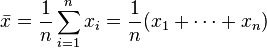Chat with us, powered by LiveChat Statistics : Mean, Mode, Median for SSC CGL Math+91-9817390373 , +91-9729327755( 9am-5pm | Mon to Sat) |[email protected]

## Statistics : Mean, Mode, Median for SSC CGL Math

Statistics : Mean, Mode, Median for SSC CGL Mathstatistics

Generally one or two questions can be asked in SSC CGL exam from statistics. However there are 4-5 questions from data interpretation in SSC CGL

tier 1 and 7-8 Questions in Tier 2 can be asked.

But here statistics portion includes arithmetic mean, Geometric mean, median, mode, variance etc.

I am providing formula which will be extremely useful and practice questions to understand the concept in a better way.

### Arithmetic mean

The most common type of average is the arithmetic mean. If n numbers are given, each number denoted by ai, where i = 1, …, n, the arithmetic mean is the sum of the ai‘s divided by n or$AM = \frac{1}{n}\sum_{i=1}^na_i = \frac{1}{n}\left(a_1 + a_2 + \cdots + a_n\right)$

The arithmetic mean, often simply called the mean, of two numbers, such as 2 and 8, is obtained by finding a value A such that 2 + 8 = A + A. One may find that A= (2 + 8)/2 = 5. Switching the order of 2 and 8 to read 8 and 2 does not change the resulting value obtained for A. The mean 5 is not less than the minimum 2 nor greater than the maximum 8. If we increase the number of terms in the list to 2, 8, and 11, the arithmetic mean is found by solving for the value of A in the equation 2 + 8 + 11 = A + A + A. One finds that A = (2 + 8 + 11)/3 = 7.

#### Geometric mean

The geometric mean of n non-negative numbers is obtained by multiplying them all together and then taking the nth root. In algebraic terms, the geometric mean ofa1a2, …, an is defined as$GM= \sqrt[n]{\prod_{i=1}^n a_i} = \sqrt[n]{a_1 a_2 \cdots a_n}$

Example: Geometric mean of 2 and 8 is$GM = \sqrt{2 \cdot 8} = 4$

#### Mode

The most frequently occurring number in a list is called the mode. For example, the mode of the list (1, 2, 2, 3, 3, 3, 4) is 3. It may happen that there are two or more numbers which occur equally often and more often than any other number. In this case there is no agreed definition of mode. Some authors say they are all modes and some say there is no mode.

#### Median

The median is the middle number of the group when they are ranked in order. (If there are an even number of numbers, the mean of the middle two is taken.)

Thus to find the median, order the list according to its elements’ magnitude and then repeatedly remove the pair consisting of the highest and lowest values until either one or two values are left. If exactly one value is left, it is the median; if two values, the median is the arithmetic mean of these two. This method takes the list 1, 7, 3, 13 and orders it to read 1, 3, 7, 13. Then the 1 and 13 are removed to obtain the list 3, 7. Since there are two elements in this remaining list, the median is their arithmetic mean, (3 + 7)/2 = 5.

## So

Arithmetic Mean$\bar{x} = \frac{1}{n}\sum_{i=1}^n x_i = \frac{1}{n} (x_1 + \cdots + x_n)$

Geometric MeanYou may like other similar important topics

### 26 Responses to “Statistics : Mean, Mode, Median for SSC CGL Math”

•freight says:

Not requiring a prescription, the Anabolic steroids can be easily purchased online.
Upon return of the defective product and verification against the RMA, the temporary charge hold on the customer’s credit card
will be lifted. However, ocean cargo shipping is typically the
more affordable of the two.

•Mayank Saxena says:

Sir can you provide solution of project 400. And also provide tricks for the each topics